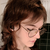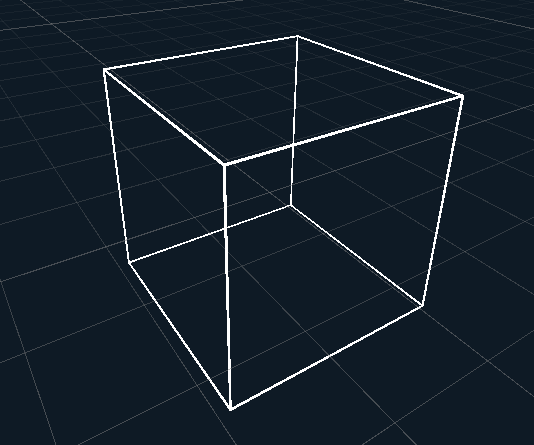# Gizmos.DrawWireCube Equivalent• updated

Hello, I have a simple question,

what is the equivalent to Gizmos.DrawWireCube or in general how can I only draw a generic wire shape such as circle and cube for example?

Reporting a bug? please specify Unity version:
Reporting a bug? please specify Shapes version:
Reporting a bug? please specify Render Pipeline:
Built-in render pipeline
Pinned repliesFreya Holmér

there are no built-in meta-shapes like this, so you'd have to write your own based on what needs you have

Drawing a wire cube for instance is a matter of iterating through all the pairs of points making up the edges of a cube and drawing a line:`// Draws a wireframe cube using Draw.Linestatic void DrawWireCube( Vector3 position, Quaternion rotation, Vector3 scale ) {   using( Draw.MatrixScope ) {      Draw.Matrix *= Matrix4x4.TRS( position, rotation, scale );      foreach( ( Vector3 a, Vector3 b ) in cubeEdges )         Draw.Line( a, b, LineEndCap.Round );   }}// an array of all vertex pairs of a cube's edgesstatic readonly (Vector3 a, Vector3 b)[] cubeEdges = GetCubeEdges().ToArray();// generates all vertex pairs of cube edgesstatic IEnumerable<(Vector3, Vector3)> GetCubeEdges() {   Vector3 Scoot( Vector3 v, int n ) => new Vector3( v[n], v[( n + 1 ) % 3], v[( n + 2 ) % 3] );   for( int a = 0; a < 3; a++ )      for( int ix = -1; ix < 2; ix += 2 )         for( int iy = -1; iy < 2; iy += 2 )            yield return (               Scoot( new Vector3( -1, ix, iy ), a ),               Scoot( new Vector3( 1, ix, iy ), a )            );}`
Replies 3Freya Holmér

there are no built-in meta-shapes like this, so you'd have to write your own based on what needs you have

Drawing a wire cube for instance is a matter of iterating through all the pairs of points making up the edges of a cube and drawing a line:`// Draws a wireframe cube using Draw.Linestatic void DrawWireCube( Vector3 position, Quaternion rotation, Vector3 scale ) {   using( Draw.MatrixScope ) {      Draw.Matrix *= Matrix4x4.TRS( position, rotation, scale );      foreach( ( Vector3 a, Vector3 b ) in cubeEdges )         Draw.Line( a, b, LineEndCap.Round );   }}// an array of all vertex pairs of a cube's edgesstatic readonly (Vector3 a, Vector3 b)[] cubeEdges = GetCubeEdges().ToArray();// generates all vertex pairs of cube edgesstatic IEnumerable<(Vector3, Vector3)> GetCubeEdges() {   Vector3 Scoot( Vector3 v, int n ) => new Vector3( v[n], v[( n + 1 ) % 3], v[( n + 2 ) % 3] );   for( int a = 0; a < 3; a++ )      for( int ix = -1; ix < 2; ix += 2 )         for( int iy = -1; iy < 2; iy += 2 )            yield return (               Scoot( new Vector3( -1, ix, iy ), a ),               Scoot( new Vector3( 1, ix, iy ), a )            );}`Works like a charm, thank you, for the reply and this awesome library.

I've been a long-time follower of you, and your math/shaders videos have helped me a lot during the years, so thank you once again.

Ps: I think you should include wire shapes in the API, I am sure I am not the only one that would love to have this feature out of the box.Freya Holmér

I'm glad you like my stuff <3

as for including wire shapes - this is unfortunately quite a lot of work, and opens up a whole can of worms of things I'd now have to add, not only for the existing shapes, but for any additional shapes I add,

not to mention, they wouldn't be able to deal with transparency as well as other shapes, since they are composite shapes

﻿